Some Notes on the Sequence Acceleration | OMICS International
Journal of Applied & Computational Mathematics

# Some Notes on the Sequence Acceleration

Faragó I*

MTA-ELTE NumNet Reseach Group, Institute of Mathematics, Eotvos Lorand University, Hungary

*Corresponding Author:
Faragó I
MTA-ELTE NumNet Reseach Group
Institute of Mathematics, Eotvos Lorand University, Hungary
Tel: +3614116500
E-mail: [email protected]

Received April 25, 2016; Accepted April 26, 2016; Published April 28, 2016

Citation: Faragó I (2016) Some Notes on the Sequence Acceleration. J Appl Computat Math 5:303. doi:10.4172/2168-9679.1000303

Copyright: © 2016 Faragó I. This is an open-access article distributed under the terms of the Creative Commons Attribution License, which permits unrestricted use, distribution, and reproduction in any medium, provided the original author and source are credited.

Visit for more related articles at Journal of Applied & Computational Mathematics

#### Opinion

In the mathematical problems we seek the solution to the problem.

F(u)=0, (1)

where F denotes some given operator (function) between two spaces. Typically we are not able to define u* in an explicit way. Therefore, by use of some numerical method, we replace (1) with the sequence of problems

Fn(un)=0, n=1,2,…, (2)

where each problem can be solved, and hence we construct the sequence To our purpose the following requirements are quite natural:

a) (un) is a convergent sequence;

b) limun=u*.

From a practical point of view, it is also important to have information about the magnitude of the global error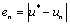, because un (for some finite n) is considered as the numerical solution, i.e., the approximation of the exact solution u*. Clearly, convergence means that en tends to zero as n approaches infinity, i.e., en is arbitrarily small for sufficiently large values of n. In order to characterize the speed of the convergence, we assume that there exists the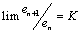. We say that the convergence is linear if K∈(0,1). When K=1, then we say that the convergence is sublinear. When K=0, the convergence is called superlinear.

For the linear convergence the rate of convergence depends on how far K is from 1: the closer K to 1, the slower the convergence. The linear convergence means that the sequence is similar to the geometric sequence. This means that the convergent sequences, based on Banach’s fixed point theorem, has linear convergence.

Therefore the linear and the sublinear convergence might be very slow, and the error quite big for some (even big) value of n. Obviously, from a practical point of view, a numerical method is efficient when the global error is small for relatively small values of n. Hence, in case of slow convergence we need to accelerate the convergence rate.

#### Aitken’s Process

We start with the example, given by the famous Japanese mathematician, Takakazu Seki, which defines an approximation to π in the following way . Let un be the perimeter of the polygon with 2n sides inscribed in a circle of diameter one. Hence

u15=3.1415926487 769856708

u16=3.1415926523 865913571

u17=3.1415926532 889927759.

Seki computed a new value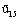as follows: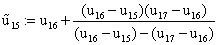(3)

which results in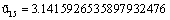.

Therefore, he concluded that

π=3.141592653589. (4)

Comparing to the exact decimal digits, we see that all the 12 digits in (4) are exact, while in the expressions for u15,u16 and u17 only the first 7, 8 and 9 digits are exact. This example shows that with a suitable operation the accuracy can be increased significantly.

The following process was suggested by Alexander Aitken .

We assume that (un) converges linearly to u*. Then, for large n we have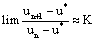and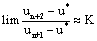(5)

Hence,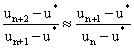(6)

which yields the relation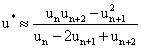(7)

Based on (7), we define the new approximation as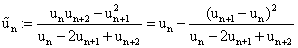(8)

Theorem: Assume that (un) converges to u* linearly. Then

a) The sequence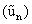converges to the same limit, i.e.,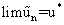(9)

b)converges faster than (un), i.e.,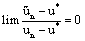(10)

Proof: Let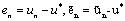, n=1,2,…. (11)

Substituting (11) into (8), we get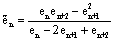(12)

In view of linear convergence, we have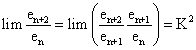(13)

Based on the identity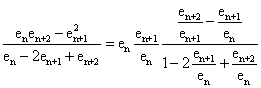(14)

which can be verified directly. Since |K|<1, for n tends to infinity (12) implies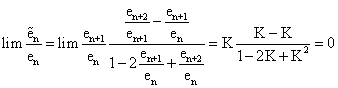(15)

which proves the statements.

We remark that that obviously the Seki’s method is an Aitken process.

#### Steffensen’s Method

The above theorem states that the convergence of the new sequence is faster than that of the original one. We can apply this approach to fixed point iterative methods for nonlinear equations. Since for this case the sequence (un) is linearly convergent, and the Aitken’s process accelerates the convergence. Namely, the correspondingsequence converges to u* linearly, but faster than (un).

We give the description of another acceleration method, which is called Steffensen’s method . Which is a good combination of the fixed point iteration and Aitken’s method .

The algorithm of this method is as follows. We set an arbitrary x0, and then x1=f(x0), x2=f(x1). Having these points, we compute by (20) the new point following Aitken’s method as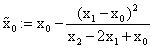,

and with this value we restart the fixed point iteration, i.e.,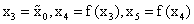.

Then we compute the approximation again by Aitken’s method as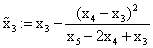,

and so on.

Steffensen’s method (similarly to Newton’s method) provides quadratic convergence. In Newton’s method we compute one function value and one derivative in each iteration step. In Steffensen’s method we have two function evaluations and a more complicated algebraic expression in each iteration, but no derivative. (However, in order to guarantee quadratic convergence for Steffensen’s method, the function f must be three times continuously differentiable, while in Newton’s method only twice.)

The following example demonstrates the methods, investigated so far.

Example: Find the unique fixed point of the function f(x)=cosx on the interval [0,1]. We use three methods :

1. Fixed point iteration: xn+1=f(xn)=cosxn.

2. Aitken’s method for the above sequence.

3. Steffensen’s method

(For each we choose x0=0.5 for the starting value) (Table 1).

1 2 3
0 0.50000 0.73139 0.73139
1 0.87758 0.73609 0.73908
2 0.63901 0.73765
3 0.80269 0.73847
4 0.69478 0.73880
5 0.76820
6 0.71917

Table 1: Decimal digits of accuracy.

The numerical experiment shows the following. To compute the fixed point to ten decimal digits of accuracy, the fixed point iteration requires 57 iterates, Aitken’s method 25 iterates, while Steffensen’s method requires only 3 iterates. (This yields that in the fixed point iteration for Aitken’s method 27 basic points, for Steffensen’s method 11 basic points are needed).

#### Richardson’s Method

Aitken’s method is applicable for the acceleration of linearly convergent sequences. But, even for this case we cannot predict the magnitude of the increase. To give the characterization of the rate of convergence, we say that a sequence (un) converges to u* with order p if there exists a constant C≥0 such that the inequality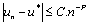holds for all, sufficiently large n. In numerical modelling, the sequence (un) is generated by solving the numerical model (2). The discrete models are typically constructed as the formulation of the model (1) on a sequence of discrete meshes (grids), and n depends on the number of grid points. Since in the discretization process the number of grid points is inversely proportional to the grid spacing parameter h, we may assume that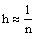.

Hence, the Landau notation can be written as O(hP), which means that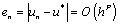(16)

This can be written as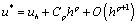(17)

The Richardson’s method based on the following observation . When we have 2 different sequences, obtained by the same numerical method but on different meshes, formula (19) gives possibility to eliminate the term Cphp, and we obtain a method of higher order. E.g., when the sequences of p-th order are generated on the meshes of the step-size 2h and h, respectively, based on the corresponding two approximations we define the new approximation as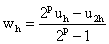, (18)

which will have the order of convergence p+1.

#### Conclusion

While the Aitken’s and Steffensen’s methods serve to accelerate the speed of the convergence of some fixed sequence by use of some transformation of the elements of this sequence, the Richardson’s method does the acceleration by use of two sequences, generated on by the same method but on different meshes.

#### References

Select your language of interest to view the total content in your interested language

### Article Usage

• Total views: 8535
• [From(publication date):
May-2016 - Dec 16, 2019]
• Breakdown by view type
• HTML page views : 8447Can't read the image? click here to refresh